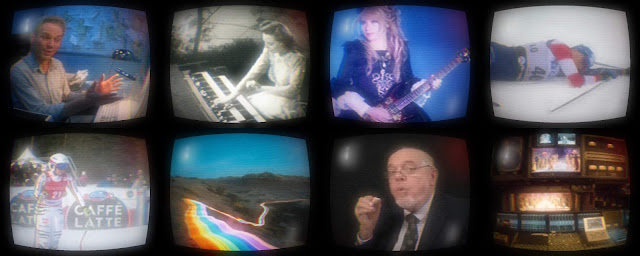### FFmpeg: CRT Screen Effect

A simple attempt at creating a [stylised] 'CRT screen' effect with FFmpeg. Loaded with the common CRT effect tropes and clichés; interlaced lines, noise, chromatic aberration, bloom etc.

The filterchains were constructed to be modular; allowing them to be included or removed, as desired. The ideas included in these filterchains might be of more use in general, than the whole effect itself.

```#!/bin/bash

# A collection of FFmpeg filterchains which can be used to create a stylised
# 'CRT screen' effect on given input.
#
# The filter-chains have been split apart to increase modularity at the cost of
# sacrificing simplicity and increasing redundant code. Filter-chains can be
# added or removed in various orders, but special attention must be paid to
# selecting the correct termination syntax for each stage.
#
# Includes basic demonstration FFmpeg command which takes "\$1" input file.
#
# Version: 2019.04.06_02.49.13
# Source https://oioiiooixiii.blogspot.com

### FILTERCHAINS #############################################################

# Reduce input to 25% PAL resolution
shrink144="scale=-2:144"

# Crop to 4:3 aspect ratio at 25% PAL resolution
crop43="crop=180:144"

# Create RGB chromatic aberration
rgbFX="split=3[red][green][blue];
[red] lutrgb=g=0:b=0,
scale=188x144,
crop=180:144 [red];
[green] lutrgb=r=0:b=0,
scale=184x144,
crop=180:144 [green];
[blue] lutrgb=r=0:g=0,
scale=180x144,
crop=180:144 [blue];
format=gbrp"

# Create YUV chromatic aberration
yuvFX="split=3[y][u][v];
[y] lutyuv=u=0:v=0,
scale=192x144,
crop=180:144 [y];
[u] lutyuv=v=0:y=0,
scale=188x144,
crop=180:144 [u];
[v] lutyuv=u=0:y=0,
scale=180x144,
crop=180:144 [v];
[y][v] blend=all_mode='lighten' [yv];
[yv][u] blend=all_mode='lighten'"

# Create edge contour effect
edgeFX="edgedetect=mode=colormix:high=0"

# Add noise to each frame of input
noiseFX="noise=c0s=7:allf=t"

# Add interlaced fields effect to input
interlaceFX="split[a][b];
[a] curves=darker [a];
[a][b] blend=all_expr='if(eq(0,mod(Y,2)),A,B)':shortest=1"

# Re-scale input to full PAL resolution
scale2PAL="scale=720:576"

# Re-scale input to full PAL resolution with linear pixel
scale2PALpix="scale=720:576:flags=neighbor"

# Add magnetic damage effect to input [crt screen]
screenGauss="[base];
nullsrc=size=720x576,
drawtext=
fontfile=/usr/share/fonts/truetype/freefont/FreeSerif.ttf:
text='@':
x=600:
y=30:
fontsize=170:
fontcolor=red@1.0,
boxblur=80 [gauss];
[gauss][base] blend=all_mode=screen:shortest=1"

# Add reflections to input [crt screen]
reflections="[base];
nullsrc=size=720x576,
format=gbrp,
drawtext=
fontfile=/usr/share/fonts/truetype/freefont/FreeSerif.ttf:
text='€':
x=50:
y=50:
fontsize=150:
fontcolor=white,
drawtext=
fontfile=/usr/share/fonts/truetype/freefont/FreeSerif.ttf:
text='J':
x=600:
y=460:
fontsize=120:
fontcolor=white,
boxblur=25 [lights];
[lights][base] blend=all_mode=screen:shortest=1"

# Add more detailed highlight to input [crt screen]
highlight="[base];
nullsrc=size=720x576,
format=gbrp,
drawtext=
fontfile=/usr/share/fonts/truetype/freefont/FreeSerif.ttf:
text='¡':
x=80:
y=60:
fontsize=90:
fontcolor=white,
boxblur=7 [lights];
[lights][base] blend=all_mode=screen:shortest=1"

# Curve input to mimic curve of crt screen
curveImage="vignette,
format=gbrp,
lenscorrection=k1=0.2:k2=0.2"

# Add bloom effect to input [crt screen]
bloomEffect="split [a][b];
[b] boxblur=26,
format=gbrp [b];
[b][a] blend=all_mode=screen:shortest=1"

### FFMPEG COMMAND ###########################################################

ffmpeg \
-i "\$1" \
-vf "
\${shrink144},
\${crop43},
\${rgbFX},
\${yuvFX},
\${noiseFX},
\${interlaceFX},
\${scale2PAL}
\${screenGauss}
\${reflections}
\${highlight},
\${curveImage},
\${bloomEffect}
" \
"\${1}__crtTV.mkv"

exit 0```